## Nikita Shah

student | Maths Chemistry Physics Biology

### Description

hi

SETS - Concept, Type of sets, Properties of Sets with Example.

SETS

A set is a collection of well defined objects which are distinct from each other.

Set are generally denoted by capital letters A, B, C, .... etc. and the elements of the set by a, b, c .... etc.
If a is an element of a set A, then we write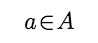and say a belongs to A.
If a does not belong to A then we write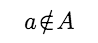.

e.g. The collection of first five prime natural numbers is a set containing the elements 2, 3, 5, 7, 11.

Some Important Number Sets:

N = Set of all natural numbers = {1, 2, 3, 4, ....}

W = Set of all whole numbers = {0, 1, 2, 3, ....}

Z or I set of all integers
= {.... –3, –2, –1, 0, 1, 2, 3, ....}

Z+ = Set of all +ve integers = {1, 2, 3, ....} = N.

Z– = Set of all –ve integers = (–1, –2, –3, ....}

Z0 = The set of all non-zero integers. = {±1, ±2, ±3, ....}

Q = The set of all rational numbers.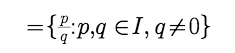R = the set of all real numbers.

R-Q = The set of all irrational numbers.

e.g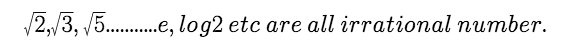METHODS TO WRITE A SET

1.Roster Method :

In this method a set is described by listing elements, separated by commas and enclose then by curly brackets

e.g. The set of vowels of English Alphabet may be described as {a, e, i, o, u}

2.Set Builder Form:

In this case we write down a property or rule p Which gives us all the element of the set.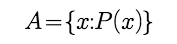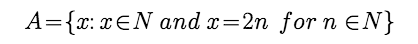I.e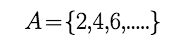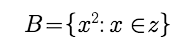i.e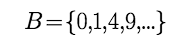TYPES OF SETS:

Null of empty sets :

A set having no element in it called an Empty Set or Null Set or Void Set. It is denoted by { }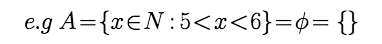A set consiting of atleast one element called a non empty set or non void set.

Example 1: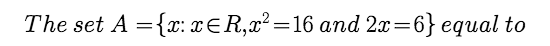Solution :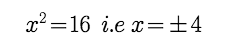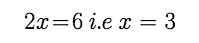So there is no value which x satisfies both the euqaution This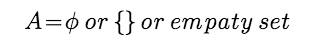Singleton : A set consisting of a single element is called a singleton set.

e.g. Then set {0}, is a singleton set

Finite Set : A set which has only finite number of elements is called a finite set.

e.g. A = {a, b, c}

Order of a finite set : The number of elements in a finite set is called the order of the set A and is denoted O(A) or n(A). It is also called cardinal number of the set.

e.g.A={a,b,c,d} n(A)=4

Infinite set : A set which has an infinite number of elements is called an infinite set.

e.g. A = {1, 2, 3, 4, ....} is an infinite set

Equal sets : Two sets A and B are said to be equal if every element of A is a member of B, and every element of B is a member of A.

If sets A and B are equal. We write A = B and A and B are not equal then A B e.g.A={1,2,6,7} and B={6,1,2,7} A=B

Equivalent sets : Two finite sets A and B are equivalent if their number of elements are same ie. n(A) = n(B)

e.g.A={1,3,5,7}, B={a,b,c,d}

n(A) = 4 and n(B) = 4 n(A) = n(B)

Note : Equal set always equivalent but equivalent sets may not be equal

Subsets : Let A and B be two sets if every element of A is an element B, then A is called a subset of B if A is a subset of B. we write A B

Example : A={1,2,3,4}andB={1,2,3,4,5,6,7} A B

Proper subset : If A is a subset of B and A B then A is a proper subset of B. and we write A B Note-1 : Every set is a subset of itself i.e. A A for all A

Point 1 Empty set is a subset of every set.

Point 2: The total number of subsets of a finite set containing n elements is 2n.

Universal set : A set consisting of all possible elements which occur in the discussion is called a Universal set and is denoted by U.

Point : All sets are contained in the universal set

e.g. If A = {1, 2, 3}, B = {2, 4, 5, 6}, C = {1, 3, 5, 7} then U = {1, 2, 3, 4, 5, 6, 7} can be taken as the Universal set.

Power set : Let A be any set. The set of all subsets of A is called power set of A and is denoted by P(A).

Example 2:

Two finite sets of have m and n elements respectively the total number of elements in power set of first set is

56 more thatn the total number of elements in power set of the second set find the value of m and n

respectively.

Solution :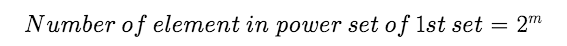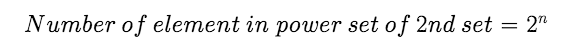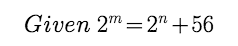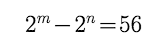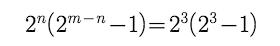so m =6 and n = 3

Example :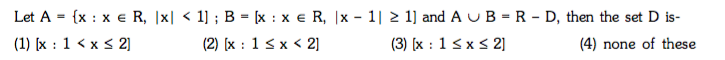Solution :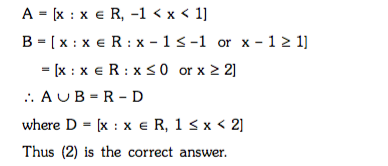Disjoint Sets :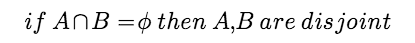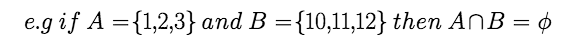Point to Note :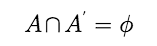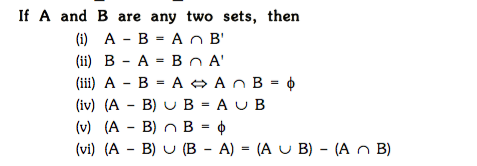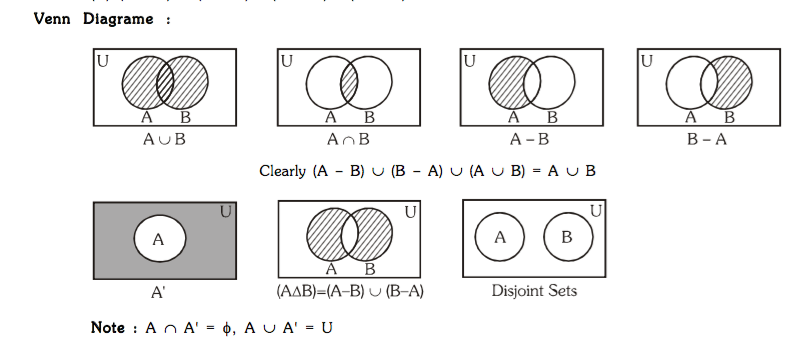STATISTICS - MEAN, MEDIAN, MODE- Concept with Example

STATISTICS

An average value of a distribution is the value of variable which is representative of the entire distribution, this representative value are called the measures of central tendency.
Generally the following five measures of central tendency.
(a) Mathematical average

(i) Arithmetic mean (ii) Geometric mean

(b) Positional average

(i) Median (ii) Mode

ARITHMETIC MEAN :

1. For ungrouped dist :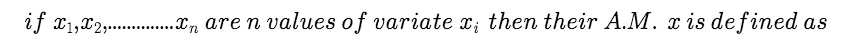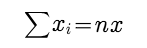2.For ungrouped and grouped freq. dist.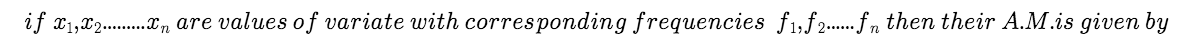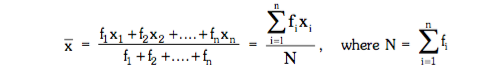No Active Challenge